# RD Sharma Solutions for Class 8 Maths Chapter - 7 Factorization Exercise 7.7

Until now, we were discussing concepts based on monomial and binomial expressions. In this exercise, we shall discuss polynomials and factorization of quadratic polynomials in one variable. The primary objective is to help students improve their problem-solving abilities, which in turn helps in boosting their confidence level to achieve high marks in their exams. Students can refer and download RD Sharma Solutions Maths Exercise 7.7 Chapter 7 Factorization from the links given below.

## Download the pdf of RD Sharma Solutions For Class 8 Maths Exercise 7.7 Chapter 7 Factorization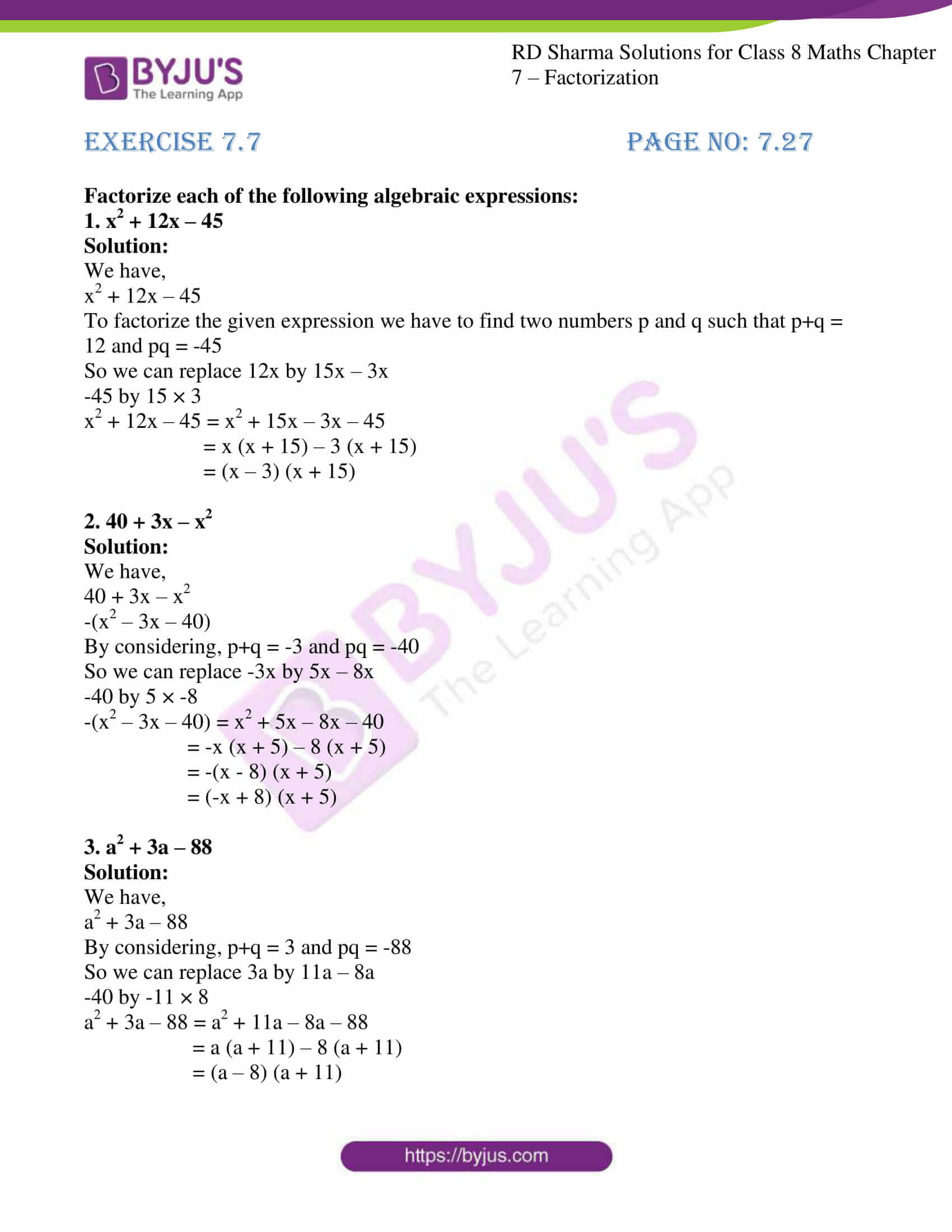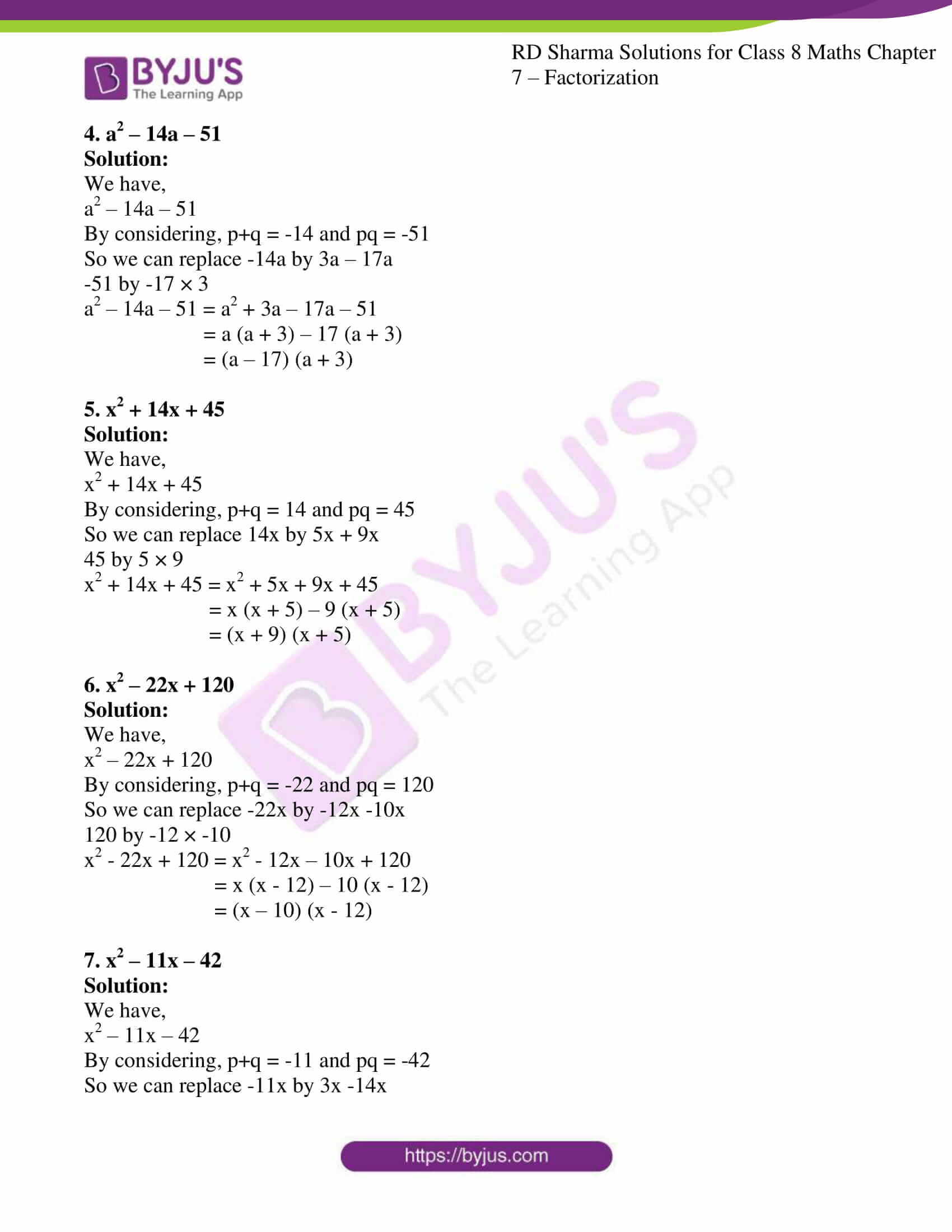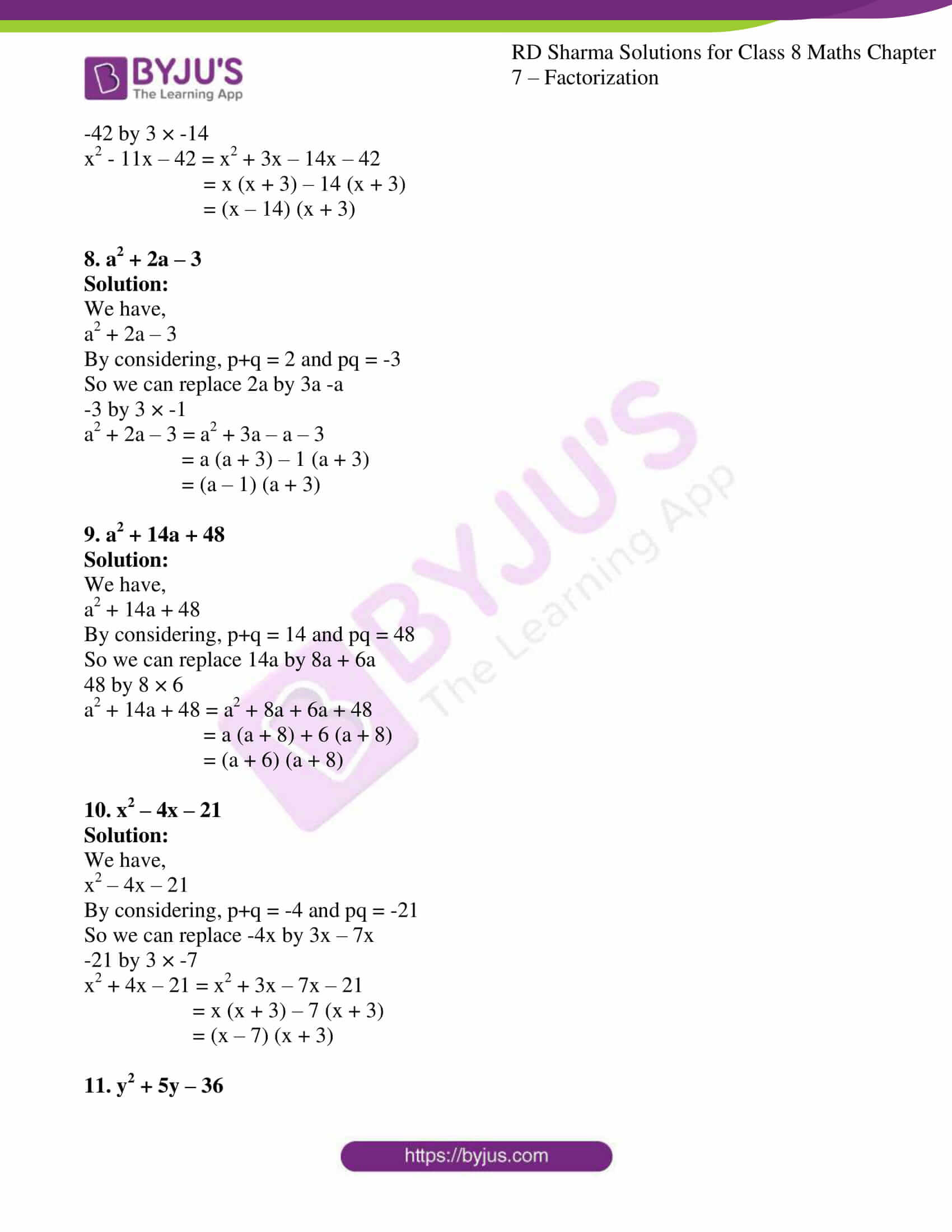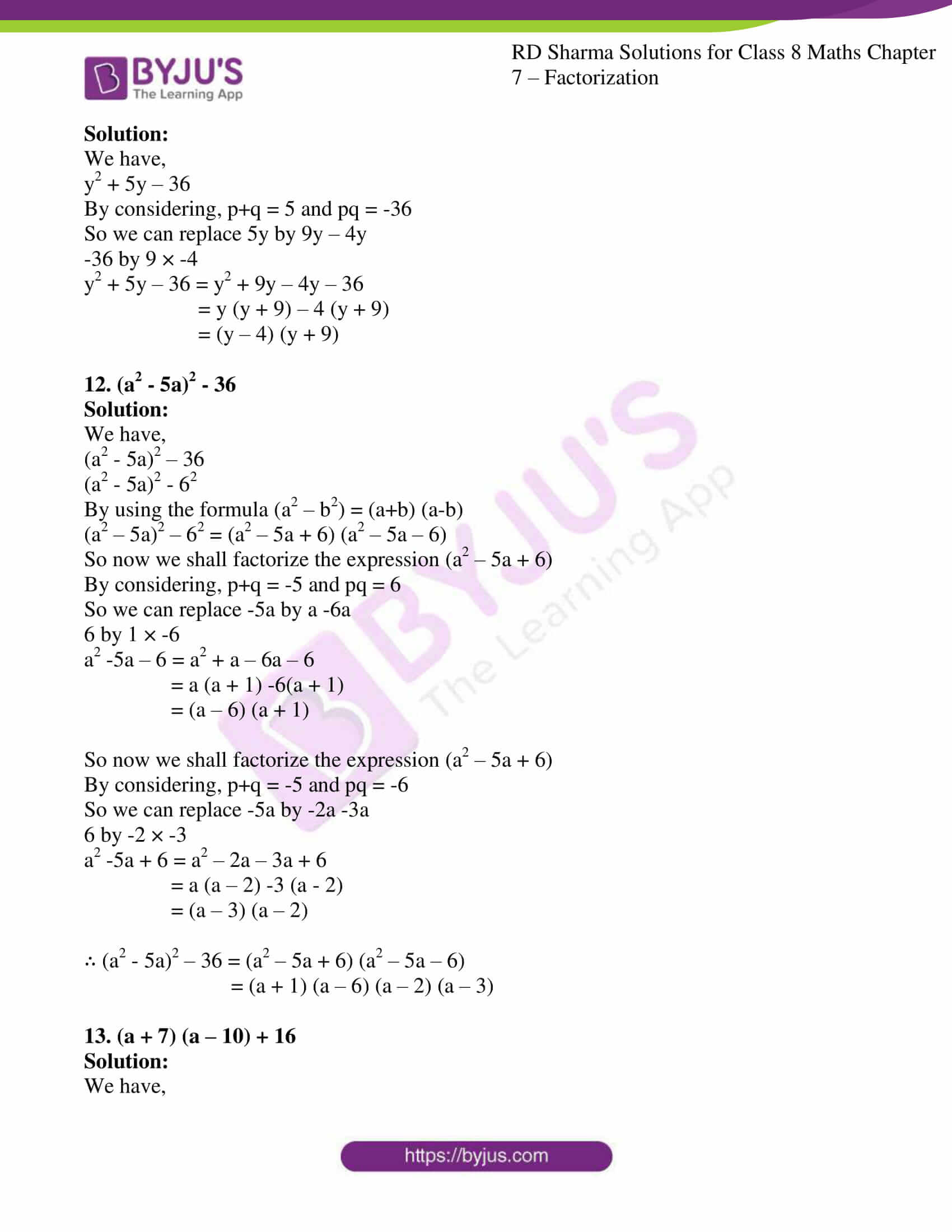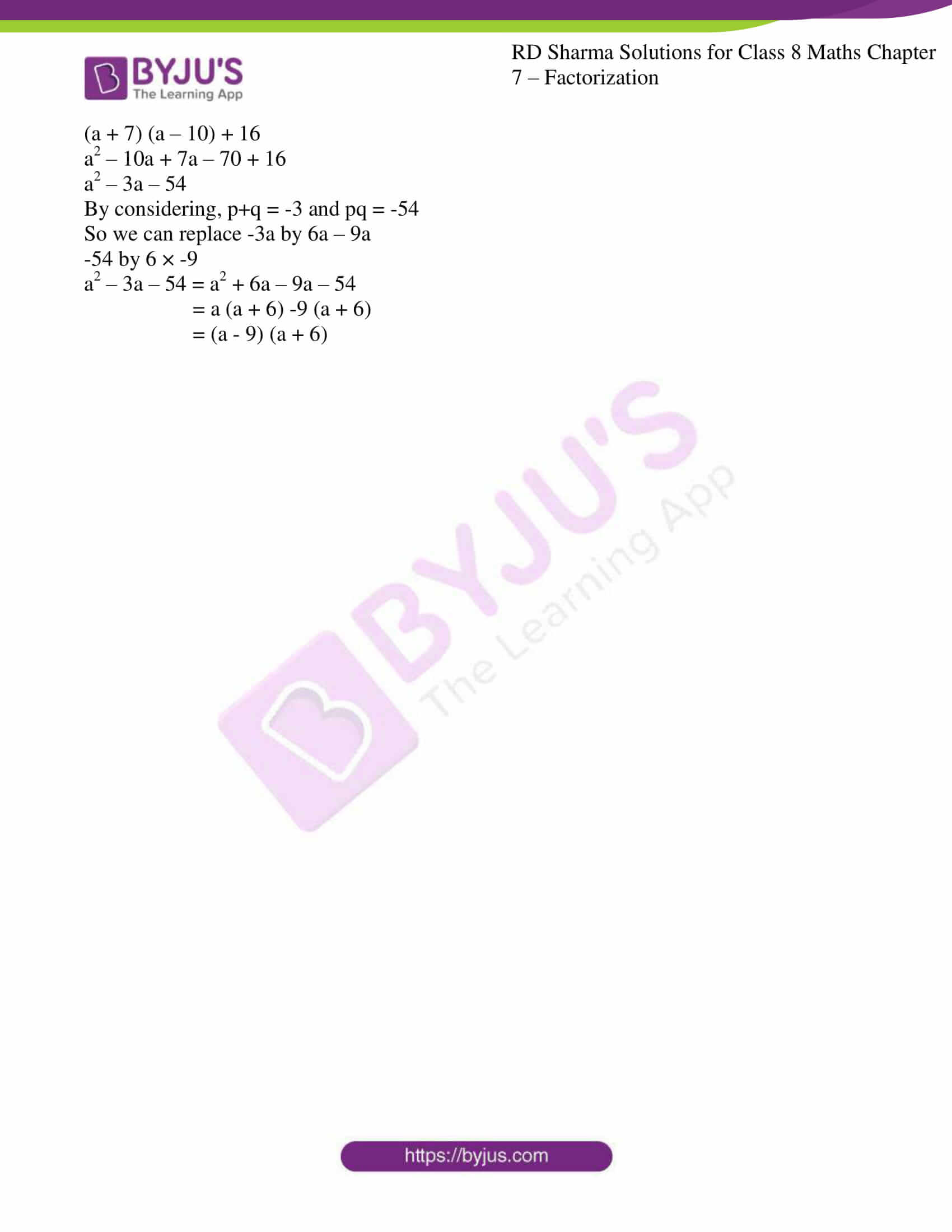### Access Answers to RD Sharma Solutions for Class 8 Maths Exercise 7.7 Chapter 7 Factorization

#### EXERCISE 7.7 PAGE NO: 7.27

Factorize each of the following algebraic expressions:

1. x2 + 12x – 45

Solution:

We have,

x2 + 12x – 45

To factorize the given expression we have to find two numbers p and q such that p+q = 12 and pq = -45

So we can replace 12x by 15x – 3x

-45 by 15 × 3

x2 + 12x – 45 = x2 + 15x – 3x – 45

= x (x + 15) – 3 (x + 15)

= (x – 3) (x + 15)

2. 40 + 3x – x2

Solution:

We have,

40 + 3x – x2

-(x2 – 3x – 40)

By considering, p+q = -3 and pq = -40

So we can replace -3x by 5x – 8x

-40 by 5 × -8

-(x2 – 3x – 40) = x2 + 5x – 8x – 40

= -x (x + 5) – 8 (x + 5)

= -(x – 8) (x + 5)

= (-x + 8) (x + 5)

3. a2 + 3a – 88

Solution:

We have,

a2 + 3a – 88

By considering, p+q = 3 and pq = -88

So we can replace 3a by 11a – 8a

-40 by -11 × 8

a2 + 3a – 88 = a2 + 11a – 8a – 88

= a (a + 11) – 8 (a + 11)

= (a – 8) (a + 11)

4. a2 – 14a – 51

Solution:

We have,

a2 – 14a – 51

By considering, p+q = -14 and pq = -51

So we can replace -14a by 3a – 17a

-51 by -17 × 3

a2 – 14a – 51 = a2 + 3a – 17a – 51

= a (a + 3) – 17 (a + 3)

= (a – 17) (a + 3)

5. x2 + 14x + 45

Solution:

We have,

x2 + 14x + 45

By considering, p+q = 14 and pq = 45

So we can replace 14x by 5x + 9x

45 by 5 × 9

x2 + 14x + 45 = x2 + 5x + 9x + 45

= x (x + 5) – 9 (x + 5)

= (x + 9) (x + 5)

6. x2 – 22x + 120

Solution:

We have,

x2 – 22x + 120

By considering, p+q = -22 and pq = 120

So we can replace -22x by -12x -10x

120 by -12 × -10

x2 – 22x + 120 = x2 – 12x – 10x + 120

= x (x – 12) – 10 (x – 12)

= (x – 10) (x – 12)

7. x2 – 11x – 42

Solution:

We have,

x2 – 11x – 42

By considering, p+q = -11 and pq = -42

So we can replace -11x by 3x -14x

-42 by 3 × -14

x2 – 11x – 42 = x2 + 3x – 14x – 42

= x (x + 3) – 14 (x + 3)

= (x – 14) (x + 3)

8. a2 + 2a – 3

Solution:

We have,

a2 + 2a – 3

By considering, p+q = 2 and pq = -3

So we can replace 2a by 3a -a

-3 by 3 × -1

a2 + 2a – 3 = a2 + 3a – a – 3

= a (a + 3) – 1 (a + 3)

= (a – 1) (a + 3)

9. a2 + 14a + 48

Solution:

We have,

a2 + 14a + 48

By considering, p+q = 14 and pq = 48

So we can replace 14a by 8a + 6a

48 by 8 × 6

a2 + 14a + 48 = a2 + 8a + 6a + 48

= a (a + 8) + 6 (a + 8)

= (a + 6) (a + 8)

10. x2 – 4x – 21

Solution:

We have,

x2 – 4x – 21

By considering, p+q = -4 and pq = -21

So we can replace -4x by 3x – 7x

-21 by 3 × -7

x2 + 4x – 21 = x2 + 3x – 7x – 21

= x (x + 3) – 7 (x + 3)

= (x – 7) (x + 3)

11. y2 + 5y – 36

Solution:

We have,

y2 + 5y – 36

By considering, p+q = 5 and pq = -36

So we can replace 5y by 9y – 4y

-36 by 9 × -4

y2 + 5y – 36 = y2 + 9y – 4y – 36

= y (y + 9) – 4 (y + 9)

= (y – 4) (y + 9)

12. (a2 – 5a)2 – 36

Solution:

We have,

(a2 – 5a)2 – 36

(a2 – 5a)2 – 62

By using the formula (a2 – b2) = (a+b) (a-b)

(a2 – 5a)2 – 62 = (a2 – 5a + 6) (a2 – 5a – 6)

So now we shall factorize the expression (a2 – 5a + 6)

By considering, p+q = -5 and pq = 6

So we can replace -5a by a -6a

6 by 1 × -6

a2 -5a – 6 = a2 + a – 6a – 6

= a (a + 1) -6(a + 1)

= (a – 6) (a + 1)

So now we shall factorize the expression (a2 – 5a + 6)

By considering, p+q = -5 and pq = -6

So we can replace -5a by -2a -3a

6 by -2 × -3

a2 -5a + 6 = a2 – 2a – 3a + 6

= a (a – 2) -3 (a – 2)

= (a – 3) (a – 2)

∴ (a2 – 5a)2 – 36 = (a2 – 5a + 6) (a2 – 5a – 6)

= (a + 1) (a – 6) (a – 2) (a – 3)

13. (a + 7) (a – 10) + 16

Solution:

We have,

(a + 7) (a – 10) + 16

a2 – 10a + 7a – 70 + 16

a2 – 3a – 54

By considering, p+q = -3 and pq = -54

So we can replace -3a by 6a – 9a

-54 by 6 × -9

a2 – 3a – 54 = a2 + 6a – 9a – 54

= a (a + 6) -9 (a + 6)

= (a – 9) (a + 6)